# 3. The Non-Inverting Amplifier

The Non-Inverting Amplifier

The op-amp can be configured to produce either an inverted or non-inverted output. In the previous section we analyzed the inverting amplifier. We now repeat the analysis for the non-inverting amplifier, as shown in Figure (5).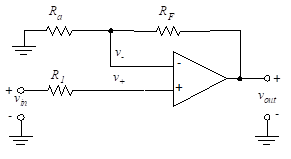Figure 5- Non-inverting amplifier

We analyze this using the basic properties of the ideal op-amp. Since the current through R1 is zero,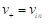(18)

Writing a node equation at the v node yields,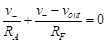(19)

We set v+ = v, and substitute for v to obtain,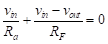(20)

The non-inverting gain is then given by,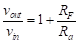(21)

We analyzed multiple-input inverting op-amps in previous Section (“The Inverting Amplifier”). We now analyze non-inverting op-amps with multiple inputs.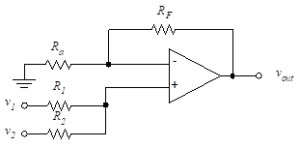Figure 6- Two non-inverting inputs

Figure (6)  illustrates a circuit with two input voltages. To find v+, we apply Kirchhoff’s current law to the non-inverting input terminal to yield (recall that the input current to the op-amp is zero),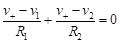(22)

Solving then for v+, we obtain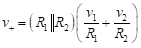(23)

The inverting voltage, v, is found from the node equation at v with the result,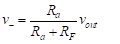(24)

Setting v+ equal to v, we obtain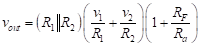(25)

###### APPLICATION

Analyze the following circuit using circuit simulation with TINACloud to determine Vout in terms of the input voltages by clicking the link below.

In a practical sense, it is important to briefly consider the effects of loading on the op-amp. The method we have been using for analysis yields the correct voltage gain,  based on the assumption that the current required from the dependent source of the ideal op-amp model is within the range set forth by the manufacturer’s data sheet for a real op-amp. Although we explore the practical considerations in later chapters, the next two examples illustrate the concept.

When analyzing both the inverting and the non-inverting configurations, we must be certain that the op-amp is capable of supplying sufficient current to drive the load resistor.

###### APPLICATIONS

Analyze the following circuits using the TINACloud online circuit simulator to determine Vout in terms of the input voltages by clicking the links below.